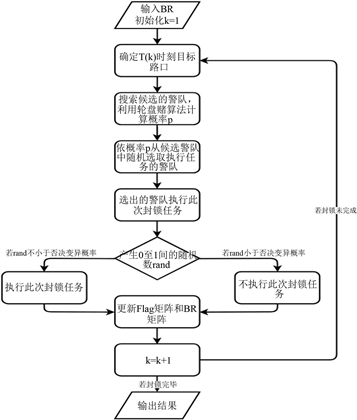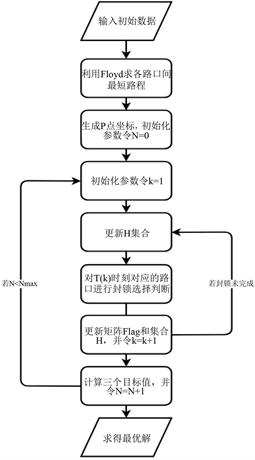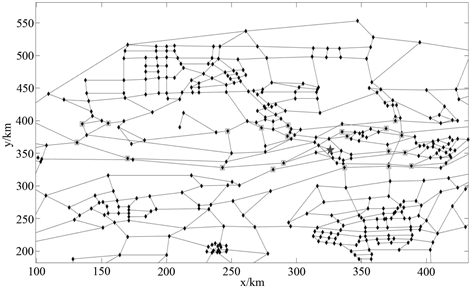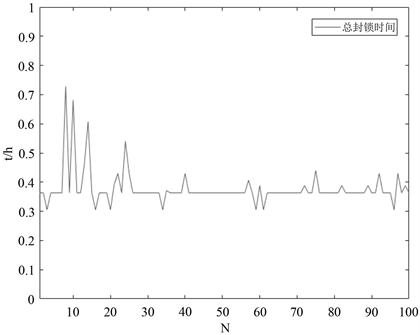# 基于改进的蚁群算法的围堵罪犯模型An Improved Ant Colony Algorithm-Based Model for Blocking Criminals

• 全文下载: PDF(862KB)    PP.762-770   DOI: 10.12677/AAM.2019.84086
• 下载量: 134  浏览量: 269

Traffic and patrol police service platforms are scattered in urban areas, which can rely on intelli-gence system to implement linkage. They not only deter criminals, but also respond quickly to sudden major criminal cases. Aiming at the problem of traffic and patrol police blocking criminals, this paper proposes a new blocking scheme, in which each police unit can block multiple intersec-tions continuously. A blockade route selection model, a blockade task completion decision model and an intersection blockade status update model are established. An improved ant colony algo-rithm is used, which adds a task rejection mutation mechanism to the common ant colony algorithm. Finally, according to the actual road situation of a certain urban area, the simulation and analysis prove the rationality of the results and the effectiveness of the algorithm.

1. 引言

2. 模型的建立

2.1. 封锁路线选择体系的建立

$R\left(t\right)={v}_{1}\cdot t$ (1)

$R\left(t\right)\le {x}_{i},\left(t\ge {t}_{c},i\in A\right)$ (2)

$\frac{{r}_{ij}\left({t}_{j1}\right)}{{v}_{2}}+{t}_{j1}\le t$ (3)

${t}_{j1}=\frac{{r}_{ij}\left({t}_{j1}\right)}{{v}_{2}}+{t}_{j1}$ (4)

2.2. 封锁任务完成的确定

$\begin{array}{l}{D}_{1}\subseteq A\\ {D}_{2}\subseteq A\\ {D}_{3}\subseteq {D}_{2}\\ {D}_{1}\cap {D}_{3}=\varphi \end{array}$ (5)

2.3. 路口状态的确定

1) 该路口已被封锁。

2) 该路口尚未被封锁。

${D}_{1}=\left\{i\in A|{\text{flag}}_{i}=2\right\}$ (6)

${\text{flag}}_{i}=0,\left(R\left(t\right)={x}_{i}\right)$ (7)

$M\left(i,{i}_{1}\right)=1,i\in {D}_{2},{i}_{1}\in {D}_{1}$ (8)

$\left\{\begin{array}{l}{\text{flag}}_{i}=2,\text{\hspace{0.17em}}\text{if}\left\{\begin{array}{l}{\text{flag}}_{i}=0\\ R\left(t\right)={x}_{i}\\ \underset{{i}_{1}}{\sum }M\left(i,{i}_{1}\right)\ge 1,\text{ }{i}_{1}\in {D}_{1}\\ i\in {D}_{2}\end{array}\\ {\text{flag}}_{i}={\text{flag}}_{i},\text{ }\text{else}\end{array}$ (9)

${D}_{3}=\left\{i\in {D}_{2}|\underset{{i}_{1}}{\sum }M\left(i,{i}_{1}\right)\ge 1,{i}_{1}\in {D}_{1}\right\}$ (10)

$\left\{\begin{array}{cc}{\text{flag}}_{i}=2& \text{if}\left\{\begin{array}{l}{\text{flag}}_{i}=0\\ R\left(t\right)={x}_{i}\\ i\in {D}_{3}\end{array}\\ {\text{flag}}_{i}={\text{flag}}_{i}& \text{else}\end{array}$ (11)

${D}_{3}={D}_{3}-\left\{i\right\},\text{ }\text{if}\text{\hspace{0.17em}}\text{\hspace{0.17em}}{\text{flag}}_{i}=2$ (12)

2.4. 目标函数求解

1) 完成所有封锁任务的总用时尽量短；

2) 封锁的区域面积尽量少；

3) 被封锁的路口数量尽量少。

$\mathrm{min}{t}_{all}$ (13)

$\mathrm{min}‖\left\{i\in A|{\text{flag}}_{i}=1\right\}‖$ (14)

$\mathrm{min}‖{D}_{1}‖$ (15)

3. 模型的求解与算法设计

3.1. 改进的蚁群算法

$n$ 个路口中去依此搜索对应路口进行的封锁，继而对罪犯完成封锁任务，这是一个 $NP$ 问题。因此在实际求解时，通常需要利用启发式算法对问题进行求解。若用简单的蚁群算法    ，则在搜索中很容易陷入对局部最优解的搜索，因此，本文采用改进的蚁群算法来对问题进行求解。我们在通常的蚁群算法中增加了任务否决变异机制，其主要思路为：当选定了某个位置的警队去执行当前路口的封锁任务时，以一定概率取消此次任务，这样就能够一定程度上避免局部搜索最优解的情况。为方便起见，之后我们称当前时刻需判断是否应被封锁的路口为目标路口。改进的蚁群算法步骤如下：

1) 初始化信息素矩阵

2) 封锁匹配选择算子：

$p\left(i,j\right)=\left\{\begin{array}{cc}\frac{BR{\left(i,j\right)}^{\alpha }\cdot {\left(1/S\left(i,j\right)\right)}^{\beta }}{\underset{i}{\sum }BR{\left(i,j\right)}^{\alpha }\cdot {\left(1/S\left(i,j\right)\right)}^{\beta }}& j\in POL\\ 0& \text{else}\end{array}$ (16)

3) 任务否决变异机制：

4) 更新路口状态：

5) 更新信息素矩阵：

$B{R}_{ij}\left(t+1\right)=B{R}_{ij}\left(t\right)\cdot \left(1-\mu \right),\left(i,j\in A\right)$ (17)

$B{R}_{ij}\left(t+1\right)=B{R}_{ij}\left(t+1\right)+\left(1-B{R}_{ij}\left(t+1\right)\right)\cdot \left(\underset{i}{\overset{3}{\sum }}{\text{goal}}_{i}\cdot {\lambda }_{i}\right)$ (18)

${\text{goal}}_{i}=\frac{{g}_{i}-\mathrm{min}{G}_{i}}{\mathrm{max}\left\{{g}_{i}+{G}_{i}\right\}-\mathrm{min}\left\{{g}_{i}+{G}_{i}\right\}}$ (19)

3.2. 警队最优封锁方案求解

Step 1：利用 $\text{Floyd}$ 算法求各路口之间的最短路程。

Step 2：随机生成 $P$ 点作为罪犯初始逃跑地点， $N=0$

Step 3：初始化参数，令 $k=1$

Step 4：计算 $P$ 点与各路口的最短路程及对应路线，存储到集合 $H$ 中。

Step 5：计算在 $H$ 条件下 $P$ 点与各个路口的距离所对应的时间，降序存储到 $T\left(k\right)$ 向量中。

Step 6：对 $T\left(k\right)$ 时刻对应的路口进行封锁任务选择。

Step 7：更新所有路口当前封锁状态，更新集合 $H$ ，令 $k=k+1$

Step 8：判断对罪犯的全部封锁任务是否全部完成，若未完成则返回Step 4。

Step 9：计算三个目标函数值，并令 $N=N+1$ ，若 $N<{N}_{\mathrm{max}}$ 则返回Step 3。

Step 10：求 $\mathrm{min}{t}_{all}$$\mathrm{min}‖{D}_{1}‖$$\mathrm{min}‖\left\{i\in A|fla{g}_{i}=1\right\}‖$Figure 1. Flow chart of improved ant colony algorithmFigure 2. Flow chart for solving optimal blockade scheme

4. 实例分析Table 1. Solution results of three objective values

1) 总封锁用时：0.3050小时

2) 封锁区域内包含的路口数：27个

3) 被封锁的路口数：21个

5. 结论Figure 3. Schematic diagram of optimal blockade schemeFigure 4. Effect of iteration number on total blockade time

  董小小, 唐棣, 魏歆, 等. 最小封堵圈的扩张算法设计[J]. 数学的实践与认识, 2014, 44(11): 185-190.  周伟刚, 冯倩倩. 基于点截集的围堵嫌犯模型[J]. 运筹与管理, 2017, 26(10): 148-152.  杨敏, 牟廉明, 吴亚军, 等. 基于闭集的犯罪嫌疑人快速围堵算法[J]. 计算机工程与应用, 2012, 48(29): 234-238.  董金哲. 罪犯围捕中的数学方法[J]. 科技创新导报, 2012(16): 255-256.  杨剑峰. 蚁群算法及其应用研究[D]: [博士学位论文]. 杭州: 浙江大学, 2007.  秦玲. 蚁群算法的改进与应用[D]: [硕士学位论文]. 扬州: 扬州大学, 2004.  段海滨, 王道波, 朱家强, 等. 蚁群算法理论及应用研究的进展[J]. 控制与决策, 2004, 19(12): 1321-1326.  孟祥萍, 王圣镔, 王欣欣. 基于蚁群算法和轮盘算法的多Agent Q学习[J]. 计算机工程与应用, 2009, 45(16): 60-62.  马振. 改进蚁群算法及其在TSP中的应用研究[D]: [硕士学位论文]. 青岛: 青岛理工大学, 2016.